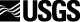## Using Visual Basic to Enhance ArcInfo GRID

### By Chip Hankley

Wisconsin Geological and Natural History Survey
Telephone: (608) 262-2320
Fax: (608) 262-8086
e-mail: dwhankley@facstaff.wisc.edu

#### ABSTRACT

Although the GRID module of ArcInfo provides a flexible modeling environment, some operations or conditions may cause extraordinarily slow GRID calculations. The multi-dimensional array capabilities of Visual Basic (VB) and Visual Basic for Applications (VBA) can mimic the raster environment of GRID, and perform spatial operations much faster. This paper demonstrates some of the performance differences between GRID and VB as well as simple techniques for transferring GRID data between ArcInfo and VB/VBA.

#### INTRODUCTION

The raster-based geographic information system, ArcInfo GRID, provides a powerful framework for model development. As models become more complex, however, GRID's execution times may become unacceptably long. When this happens, executing the most time-consuming spatial operations outside GRID may provide better results. Any computer programming language that implements multi-dimensional arrays can reproduce the spatial analysis performed in GRID -- examples include, but are not limited to, C, C++, and Visual Basic (VB).

#### WHY VISUAL BASIC AND VISUAL BASIC FOR APPLICATIONS?

Visual Basic, as opposed to C or C++, is relatively easy to learn. It represents the evolution of the BASIC language that was first introduced in 1963 (Craig and Webb, 1997). Visual Basic for Applications (VBA) represents a specific implementation of VB.

Visual Basic for Applications comes bundled with many new software packages; it essentially provides an environment for software customization using the VB language. For example, to customize Microsoft Excel, you would write a series of VB commands in the VBA editor. The main differences between VB and VBA are:

• You cannot build an executable program for distribution with VBA.
• An application written in VBA would typically have references in it to some specific component of the software in which the application is embedded. For example, an Excel VBA application would most likely refer to the worksheet names of the workbook, and thus would be meaningless if not directly attached to that Excel workbook.

Many commands work in VBA and VB, so a piece of code that you write to work in a VBA module embedded in an application will, in many cases, work in a stand-alone VB program. For example, you might develop some code using Excel to leverage the power of a spreadsheet for viewing intermediate data output; you would strip out the spreadsheet references upon completion and copy the code into VB for the final product. The choice of whether to use VBA or VB depends on the overall needs of the application. The commands and methods that this paper covers work in both environments (except where noted). For the remainder of the paper, I will only refer to VB, assuming the reader understands that for the techniques discussed, the two terms are interchangeable.

#### VISUAL BASIC ARRAYS

An array is "an ordered collection of data contained in a variable and referenced by a single variable name. Each element of the array can be referenced by a numerical subscript" (MSDN, 1998). In VB, arrays are referred to by their name, followed by a numerical reference in parentheses. The following code demonstrates how to create an array called Apple:

```	Dim Apple(4) As String 	 'Declare 	  an Array called Apple
`The array will have 4 elements
`The 4 elements will be of the 		  String data type
Apple(0) = "core"
Apple(1) = "skin"
Apple(2) = "pulp"
Apple(3) = "stems"
```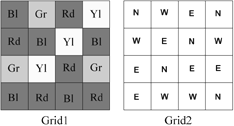Figure 1. Two hypothetical grids.

If I refer to Apple(2) later in the code, VB will return the string "pulp." Note that by default, VB numbers arrays using a base 0 format; this means that the first element in the array is numbered 0. This can be changed to base 1 by inserting the Option Base 1 statement in the general declarations section of your form or module. For the remainder of this paper, I will refer to arrays as base 1.

The previous example demonstrated a one-dimensional array; that is, the list of elements only extends in one direction. Raster data is more appropriately suited to multi-dimensional arrays.

The following code demonstrates how we might incorporate Grid1, shown in Figure 1, into an array called MyArray:

```	Dim MyArray(4, 4) As String

MyArray(1,1) = "Blue"
MyArray(1,2) = "Green"
MyArray(1,3) = "Red"
...etc.
```

In this example, I am referring to location (1, 1) as the upper left corner, with the first array element referencing rows, and the second element referencing columns. If I wanted to incorporate Grid2 into the array, I could add a 3rd dimension:

```	Dim MyArray(4, 4, 2) As String

MyArray(1, 1, 1) = "Blue"
MyArray(1, 2, 1) = "Green"
MyArray(1, 3, 1) = "Red"
...and
MyArray(1, 1, 2) = "N"
MyArray(1, 2, 2) = "W"
MyArray(1, 3, 2) = "E"
...etc.
```

Your code will be much more readable if you begin to use variables to refer to different parts of the array:

```	Dim MyArray(4, 4, 2) As String
Dim Grid1 as Integer, Grid2 as 		  Integer

Grid1 = 1
Grid2 = 2

MyArray(1, 1, Grid1) = "Blue"
...and
MyArray(1, 1, Grid2) = "N"
...etc.
```

#### MIMICKING GRID FUNCTIONS

The following GRID statement returns a value of '1' if the cell in Grid1 has a value of 'Red' and the cell in Grid2 has a value of 'N':

```	Grid3 = con(Grid1 eq "Red" AND 		Grid2 eq "N", 1, 0)
```

The following VB code would yield the same results:

```	Dim MyArray(4, 4, 3) As String
Dim Grid1 As Integer, Grid2 As 		  Integer, Grid3 As Integer
Dim x As Integer, y As Integer

Grid1 = 1
Grid2 = 2
Grid3 = 3

<...code to populate the array 		with values for Grid1 and Grid2>
For x = 1 To 4
For y = 1 To 4
If MyArray(x, y, Grid1) = 			"Red" And
MyArray(x, y, Grid2) 				= "N" Then
MyArray(x, y, Grid3) 				= "1"
Else: MyArray(x, y, Grid3) = 		"0"
End If
Next y
Next x
```

In all these examples, I am using string values to reflect the values of grid cells. Data that you import from ArcInfo will be numeric; therefore, the arrays that you declare will be of some numeric type. Visual Basic supports a variety of numeric types, including integer, long integer and single and double precision. Each data type takes up a different amount of memory. It is beyond the scope of this paper to delve into memory management in VB; however, this is an area in which to exercise some caution. If you were to import a 10 x 10 integer grid into an array and declare its type as double, the array's size would be 4 times larger than if you had declared its type as integer. Failure to effectively manage variable memory can quickly lead to 'out of memory' errors.

#### DATA EXCHANGE BETWEEN GRID AND VB

Importing data into VB from an ArcInfo raster data set involves VB code that reads the ASCII file created by the ArcInfo GRIDASCII command. Subsequently, returning data from VB to the ArcInfo environment entails VB code that writes the data from a VB array into an ASCII file of the format that the ASCIIGRID command can accept.

Below, I describe the process of importing a 4-cell by 4-cell raster dataset called Grid1 into VB. At each point in the process, I will explain the VB commands and functions that are being used.

STEP 1. Export GRID Data to an ASCII File

From GRID, issue the following command,

```	grid1.grd = GRIDASCII(Grid1)
```

This will generate a file that looks something like this:

```	ncols			4
nrows			4
xllcorner		652029.9375
yllcorner		391156.78125
cellsize		50
NODATA_value	-9999
45  55  67  78
23  3  45 6
66  8  99  12
25  37  105  44
```

STEP 2. Import Data from the ASCII File into a VB Array

In VB, begin entering code in the Form_load procedure. In VBA, you would enter code into a module.

2a) Create a variable that will return the executable file's path by using the path property.

```	Dim path As String
path = App.path & "\"
```

This is one area in which VB and VBA differ. The App object does not exist in some VBA environments. In Excel you would use the ActiveWorkbook object instead. Note that in either case, you should save your work first, so that the path property returns a path other than the default system path.

2b) Create a FileSystemObject object that will allow you to open a text file. A FileSystemObject is a VB object that allows many types of interactions with ASCII files. Use the OpenTextFile method to open the grid1.grd text file.

```	Dim f1 As Variant, in_file As 		  Variant
Set f1 = CreateObject 				  ("Scripting.FileSystemObject")
Set in_file = f1.openTextFile 		  (path + "grid1.grd")
```

2c) Create variables of the appropriate type for the six header fields. Use the readline method, combined with the mid function to return the portion of each header line that is a data (not label) element. The readline method 'reads an entire line (up to, but not including, the newline character) from a TextStream file and returns the resulting string' (MSDN, 1999). The TextStream file is the file you opened with the openTextFile method. Each time the readline method is used, VB automatically advances to the next line in the file. The mid function allows you to return characters from a string starting at a specific point. Note the line below that begins with "width." This refers to the header line "ncols 4"; position 6 is the first position past the label (ncols). In this case, the mid function will return the number 6. Finally, use the Cint (change to Integer) or CSng (Change to Single) function to convert the text string that is returned into the appropriate variable type.

```	Dim width As Integer, height As 			Integer, xll As Single, yll 		As Single
Dim CellSize As Single, NoDataSym 		As Single

```

2d) Declare a dynamic array (a dynamic array is an array that you declare without any dimensions) by using the Dim statement followed by an array name with empty parentheses after it. Re-dimension its properties to those of the input grid using the ReDim statement. Using dynamic arrays is useful when your array sizes have the possibility of changing between program instances.

```	Dim Grid1() As Integer
ReDim Grid1(width, height) As 			Integer
```

2e) Create two string variables (to represent the x and y directions on the grid) and a dynamic string array. Nest two For...Next loops, the outer one to count each row and the inner one to count each column. At the beginning of the outer loop, use the readline method in conjunction with the split function to populate the dynamic string array you just declared. The split function "returns a zero-based, one-dimensional array containing a specified number of substrings" (MSDN, 1998). You must specify what delimits the values in the string returned by readline and how many values you want to return; a -1 indicates that all substrings are returned (MSDN, 1999). Inside the inner loop, iteratively assign the individual elements of the string array to the dynamic array you created in the previous step (Grid1()).

```	Dim x As Integer, y As Integer
Dim line1() As String
For y = 1 To height
line1 = Split(in_file.readline, 		" ", -1)
For x = 1 To width
Grid1(x, y) = line1(x - 1)
Next x
Next y
```

STEP 3 Returning Data from VB to ArcInfo

Getting data out of VB and into ArcInfo basically entails reversing the above process.

3a) Use the CreateTextFile method to create a new text file (in this case called junk.grd).

```	' ---------- out_fileput to test file
Dim out_file As Variant
Set out_file = f1.CreateTextFile 		  (path + "junk.grd", True)
```

3b) Use the WriteLine method to write out the six lines of standard GRID header information. WriteLine, as the name suggests, simply writes a line of text. Each time the command is issued, VB automatically starts at the next line in the file.

```	out_file.WriteLine ("ncols " + 				CStr(width))
out_file.WriteLine ("nrows " + 				CStr(height))
out_file.WriteLine ("xllcorner " + 			CStr(xll))
out_file.WriteLine ("yllcorner " + 			CStr(yll))
out_file.WriteLine ("cellsize " + 			CStr(CellSize))
out_file.WriteLine ("NODATA_value " 			+ CStr(NoDataSym))
```

3c) Declare a one-dimensional array with the width of your grid as its number of elements. As before, nest two For...Next loops, one for rows and one for columns. In the inner loop, add data from your array to the one-dimensional array you just created. At the end of the outer loop, use the Join function to join all elements of the one-dimensional array into one text stream. If no delimiter is specified when using the Join function, a space is used (MSDN, 1999).

```	Dim line_o() as String
ReDim line_o(width)
For y = 1 To height
For x = 1 To width
line_o(x) = Grid1(x, y)
Next x
out_file.WriteLine (Join(line_o))
Next y
```

3d) Finally, close the text file.

```	out_file.Close
```

At this point, you have imported the ASCII file grid1.grd, read the file into a VB array, then written it out to an ASCII file called junk.grd. Presumably, between steps 2 and 3, you would write VB code to perform your spatial analysis, which would result in the array that you would write out in step 3.

#### VISUAL BASIC VS. GRID: COMPARING PERFORMANCE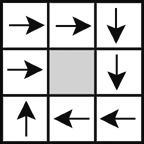Figure 2. A 3 x 3 grid with spiraling flowpath that terminates in the center.
I developed a routine that could easily be written either solely in Arc Macro Language (AML), or using a combination of AML and VB. The routine iteratively routes the cell values of an initial grid over a surface, applying a simple function to the values as they move from cell to cell (this would be similar to routing water over a topographic surface using a runoff coefficient to determine how much water passes from one cell to the next). The routine uses a spiraling flow-path that terminates in the center of the grid (figure 2). This simulates an extreme routing scenario. For the value in the upper left hand cell to reach the center, it must pass through every cell in the grid. Although this routing scenario would rarely be encountered in a real application, it provides a good scenario for comparing VB and GRID.

To compare performance, I wrote separate AMLs. The first AML utilizes DOCELL loops to accomplish the iterative routing; the second AML begins by exporting the input grids to ASCII files and then calls a VB executable that performs the routing using arrays and writes out a GRID compatible ASCII file. The final portion of this second AML imports the output grid. Appendix 1 contains the AML-only version; Appendix 2 contains the combined AML - VB version.

I created input grids of various sizes and ran the two AMLs against each of the input grids. Table 1 and figure 3 show each AML's program execution time for each grid used. Execution times were generated using ArcInfo's performance timer, which records time in one-second intervals. Some of the program execution times were too short to be accurately reflected by a one-second interval; to account for this, figure 3 shows a 0.5 second error associated with each point.

Table 1 portrays program execution time in two different ways (T1 and T2). T1 simply reflects the time it took for the program to execute; T2 attempts to look at the most time consuming process in the sample routine -- the iterative routing. T2 is shown as a range to account for any uncertainty associated with the one-second time interval, and was calculated by dividing (T1 + 0.5) by the number of cells in the input grid, and the number of iterative loops.

Table 1. AML only (GRID) and combined AML / VB (VB) performance times for the test routine.
T1 is the total program execution time in seconds; T2 is an estimate of the cell processing time
(expressed as a range) in milliseconds for the iterative phase. Where VB returned a value of
0 for T1, a time of 0.01 seconds was used in order to facilitate calculations.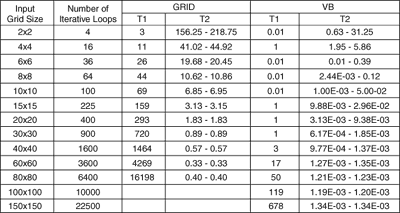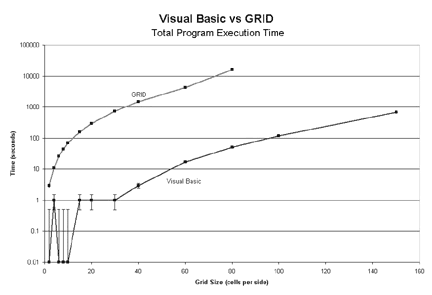Figure 3. GRID and VB performance (Table 1, T1) for a variety of input grid sizes.
The error bars show a + 0.5 second confidence.

T2 represents an attempt to quantify how long it takes for the processor to analyze one cell in the input grid. For this routine, there are many program operations that could be considered overhead. Some of these might take the same amount of time regardless of the input grid size (for example, calls to the system clock), while others may vary with input grid size (for example, ASCIIGRID and GRIDASCII commands). At some point in the program's execution, however, the processor must begin the onerous task of analyzing each cell in the input grid, moving values from cell to cell -- looping through this process until all of the values have been routed to the center. As mentioned above, the routine was set up so that the number of times the program would have to loop through this iterative process would be equal to the number of cells in the input grid. As the size of the input grid increases, the effect of the overhead processes on the overall program execution time should decrease (indicated by T2 reaching a steady state), and you should be able to compare single cell processing time between VB and GRID. Figure 4 shows that T2 seems to level off at about 0.5 milliseconds for GRID, and about 0.001 milliseconds for VB.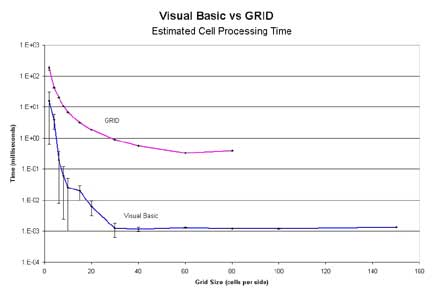Figure 4. Plot of the mean of the T2 range for each input grid size.
The error bars indicate the upper and lower bounds of the range.

On the basis of these results, it is clear that, at least for this particular type of analysis, VB far outperforms GRID: for the same numerical operation, VB may perform up to 500 times faster than GRID.

These tests were run on an Omni-Tech desktop PC, with a Pentium III 500 MHz processor and 256 MB RAM. ArcInfo 8.0.1 and Visual Basic 6.0 were used to complete the analysis.

#### CONCLUSIONS

Although it is impossible to make a sweeping comparison between the two, Table 1 and Figures 3 and 4 show that VB is much faster than GRID for the conditions tested. However, there are most likely circumstances in which GRID would outperform VB. All analyses have unique circumstances; thus the decision to use VB over GRID will rely on weighing a variety of pros and cons. Probably the most important factor to consider is the increased overhead involved in implementing a spatial project in VB over GRID (data must be imported and exported, spatial functions must be defined)--essentially you would consider if the time savings you will see by converting to VB will offset the increased development time. In those cases in which it makes sense to do spatial analyses outside of the GRID environment, VB's ease of programming its speed make it a powerful tool for the GRID modeler.

#### REFERENCES

Craig, John C., and Webb, Jeff, 1997, Microsoft Visual Basic 5.0, Developer's Workshop (Fourth Edition): Microsoft Press, Redmond, WA.

MSDN Library, Visual Studio 6.0, 1998 [Computer Program], available from: Microsoft Corporation, Redmond, WA.

#### APPENDIX 1

```/*------------------------------
/* AML_DEMO.aml
/*
/*This is a routing simulation program used to provide a testing
/*benchmark between Visual Basic and ArcInfo GRID. This version
/*uses only AML.
/*
/*REQUIRED INPUTS
/*
/*FL_DIR: a directional grid of the type created by the GRID
/*command
/*FLOWDIRECTION. Direction should be such that all cells in the
/*grid flow into and terminate at one interior cell
/*
/*RO_COEFF: a grid of values greater than or equal to 1.
/*
/*RESULTS.DAT an empty or existing text file
/*
/*THIS PROGRAM MUST BE RUN FROM GRID
/*-------------------  Program Setup
&severity &error &routine bailout
&messages &off
verify off

/*-------------------  Cleanup any Pre-existing files
&if [EXISTS t_w.grd -file] &then &sys del t_w.grd
&if [EXISTS cum_max.dat -file] &then &sys del cum_max.dat
&if [EXISTS t_w -grid] &then kill t_w all
&if [EXISTS water -grid] &then kill water all
&if [EXISTS roin -grid] &then kill roin all
&if [EXISTS fl_dir.grd -file] &then &sys del fl_dir.grd
&if [EXISTS ro_coeff.grd -file] &then &sys del ro_coeff.grd

/*-------------------  Set initial Time variable
&sv time_beg = [show &pt time]

/*----------- Create an initial grid of values to be routed
setwindow fl_dir
setcell fl_dir
water = 2 * ro_coeff
t_w = water /*Total Water Grid

/*-------------------  Set some variables
&describe water
&sv mn = %grd\$mean%
&sv cum_max = 0
&sv count = 0

/*-------------------  ROUTE the values in the water grid until
/*                     there is no water left (i.e. until it has
/*                     all flowed into the terminal cell

&do &until %mn% lt 0.000001

DOCELL
outval = scalar(0.0)
sum = scalar(0.0)
if (fl_dir(1,0) == 16) outval += water(1,0)
if (fl_dir(1,1) == 32) outval += water(1,1)
if (fl_dir(0,1) == 64) outval += water(0,1)
if (fl_dir(-1,1) == 128) outval += water(-1,1)
if (fl_dir(-1,0) == 1) outval += water(-1,0)
if (fl_dir(-1,-1) == 2) outval += water(-1,-1)
if (fl_dir(0,-1) == 4) outval += water(0,-1)
if (fl_dir(1,-1) == 8) outval += water(1,-1)
else outval += 0
ROin = float(outval)
END

water = float(ROin)
t_w = t_w + water
&describe t_w
&if %cum_max% lt %grd\$zmax% &then
&sv cum_max = %grd\$zmax%
&describe water
&sv mn = %grd\$mean%
water = water * ro_coeff
&sv count = %count% + 1

&end

/*-------------------  Set Final Time variable
&sv time_end = [show &pt time]

/*-------------------  The following lines will write out the
/*                     results of this run to a text file called
/*                     results.dat. The results written will be
/*                     1) Input Grid Size 2) Program execution
/*                     time,
/*                     3)Number of loops, and 4) Amount of 'water'
/*                     routed to the terminal cell
&describe fl_dir
&sv string = [QUOTE AML SIZE: %grd\$ncols% TIME: %time_end% LOOPS: %count% AMT: %cum_max%]

&sv open_file = [OPEN results.dat Openstat -APPEND]
&if [WRITE %open_file% %string%] <> 0 &then
&do
&type Unable to write to file
&sv close_stat = [CLOSE %open_file%]
&call exit
&end
&sv close_stat = [CLOSE %open_file%]

/*-------------------  Type the results to the screen
&type AML records that the total water collected is %cum_max%
&type    This AML did %count% loops
&type    Elapsed Program Time: %time_end% seconds
kill (!t_w water roin!) all

&call exit
&return
/********************************************
/*EXIT
&routine exit
&watch &off
&echo &off
&messages &on
&return
/* Perform Cleanup actions if Program Fails
&routine bailout
&severity &error &fail
&call exit
&return &error Bailing out of RO.aml
```

#### APPENDIX 2

There are two parts to the combined AML -- VB simulation: the AML, and the VB executable that it calls. AML portion

```/*------------------------------
/* VB_DEMO.aml
/*
/*This is a routing simulation program used to provide a testing
/*benchmark between Visual Basic and ArcInfo GRID. This version
/*utilizes a combination of VB and AML.
/*
/*REQUIRED INPUTS
/*
/*FL_DIR: a directional grid of the type created by the
/*GRID command
/*FLOWDIRECTION. Direction should be such that all cells
/*in the grid flow into and terminate at one interior cell
/*
/*RO_COEFF: a grid of values greater than or equal to 1.
/*
/*RESULTS.DAT an empty or existing text file
/*
/*THIS PROGRAM MUST BE RUN FROM GRID
/*-------------------  Program Setup
&severity &error &routine bailout
&messages &off
verify off

/*-------------------  Cleanup any Pre-existing files
&if [EXISTS t_w.grd -file] &then &sys del t_w.grd
&if [EXISTS cum_max.dat -file] &then &sys del cum_max.dat
&if [EXISTS t_w -grid] &then kill t_w all
&if [EXISTS water -grid] &then kill water all
&if [EXISTS roin -grid] &then kill roin all
&if [EXISTS fl_dir.grd -file] &then &sys del fl_dir.grd
&if [EXISTS ro_coeff.grd -file] &then &sys del ro_coeff.grd

/*-------------------  Set initial Time variable
&sv time_beg = [show &pt time]

/*-------------------  Write the input grids to ASCII files
ro_coeff.grd = gridascii(ro_coeff)
fl_dir.grd = gridascii(fl_dir)
/*-------------------  Execute the VB program
&sys vbdocell.exe

/*-------------------  Import the TOTAL WATER grid
t_w = asciigrid(t_w.grd, float)

/*-------------------  Read the cum_max  and count variables
/*                     from the cum_max.dat text file
&sv open_file = [OPEN cum_max.dat Openstat -READ]
&sv close_stat = [CLOSE %open_file%]

/*-------------------  Set Final Time variable
&sv time_end = [show &pt time]

/*-------------------  The following lines will write out the
/*                     results of this run to a text file called
/*                     results.dat. The results written will be
/*                     1) Input Grid Size 2) Program execution
/*                     time,
/*                     3)Number of loops, and 4) Amount of 'water'
/*                     routed to the terminal cell
&describe fl_dir
&sv string = [QUOTE VB SIZE: %grd\$ncols% TIME: %time_end% LOOPS: %count% AMT: %cum_max%]

&sv open_file = [OPEN results.dat Openstat -APPEND]
&if [WRITE %open_file% %string%] <> 0 &then
&do
&type Unable to write to file
&sv close_stat = [CLOSE %open_file%]
&call exit
&end
&sv close_stat = [CLOSE %open_file%]

/*-------------------  Type the results to the screen
&type VB records that the total water collected is %cum_max%
&type    Elapsed Program Time: %time_end% seconds
&type    The VB Script did %count% loops

&call exit
&return
/********************************************
/*EXIT
&routine exit
&watch &off
&echo &off
&messages &on
&return
/* Perform Cleanup actions if Program Fails
&routine bailout
&severity &error &fail
&call exit
&return &error Bailing out of RO.aml

VB Executable
You should copy this code directly into a form's code window.
Option Explicit 'Force Explicit declaration of variables
Option Base 1   'Force Base 1 arrays
'----------------------------------------------
' VB_DOCELL
'
' This is a routing simulation program used to provide a testing
' benchmark between Visual Basic and ArcInfo GRID. This VB program
' will be called from the vb_demo AML.
'
' This program demonstrates how to read in ASCII grid datasets,
' how to perform spatial operations within the VB environment, how
' to pass variables back into AML, and how to write VB arrays out
' to an ASCII format that GRID can read.
'
' REQUIRED INPUTS
'
' FL_DIR: an ASCII version of a directional grid of the type
' created by the GRID command FLOWDIRECTION. Direction should be
' such that all cells in the grid flow into and terminate at one
' interior cell.
'
' RO_COEFF: an ASCII grid of values greater than or equal to 1.
'
'----------------- Set the path for the application
Dim path As String
path = App.path & "\"
'Use the next line if working in EXCEL, comment out the previous
' one.
'path = ActiveWorkbook.path & "\"
'----------------- Open up the fl_dir and ro_coeff ascii grids
'                  and read them into an array
Dim f1 As Variant, fl_dir_file As Variant, ro_coeff_file As Variant
Set f1 = CreateObject("Scripting.FileSystemObject")
Set fl_dir_file = f1.openTextFile(path + "fl_dir.grd")
Set ro_coeff_file = f1.openTextFile(path + "ro_coeff.grd")
Dim width As Integer, height As Integer, xll As Single, yll As
Single
Dim CellSize As Single, NoDataSym As Single, c As Integer

'Skip the first 6 lines on the ro_coeff_file so that
'readline is pointing to the same location for both
For c = 1 To 6
Next c
'----------------- Read in the flow direction and ro_coeff data
' from the ASCII files
Dim FL_DIR() As Integer, ro() As Double, line1() As String
Dim line2() As String, water As Integer, ro_in As Integer
Dim t_w As Integer, RO_COEFF As Integer, x As Integer, y As
Integer

ReDim FL_DIR(width, height) As Integer
ReDim ro(width, height, 4) As Double

water = 1
ro_in = 2
t_w = 3
RO_COEFF = 4

For y = 1 To height
line1 = Split(fl_dir_file.readline, " ", -1)   'flow direction
line2 = Split(ro_coeff_file.readline, " ", -1) 'ro_coeff
For x = 1 To width
FL_DIR(x, y) = line1(x - 1)
ro(x, y, RO_COEFF) = line2(x - 1)
Next x
Next y
'Close the input files
fl_dir_file.Close
ro_coeff_file.Close

'----------------- Populate the ro_array with an initial value
For y = 1 To height
For x = 1 To width
ro(x, y, water) = 2 * ro(x, y, RO_COEFF)
ro(x, y, t_w) = ro(x, y, water)
Next x
Next y
'----------------- Begin routing the water
Dim sum As Double, cum_max As Double, Count As Integer

Count = 0
cum_max = 0
sum = 1
Do While sum > 0
For y = 1 To height
For x = 1 To width
If FL_DIR(x, y) = 1 And x <> width Then
ro(x + 1, y, ro_in) = ro(x + 1, y, ro_in) +
ro(x, y, water)
End If
If FL_DIR(x, y) = 2 And x <> width And y <> height Then
ro(x + 1, y + 1, ro_in) = ro(x + 1, y + 1,
ro_in) + ro(x, y, water)
End If
If FL_DIR(x, y) = 4 And y <> height Then
ro(x, y + 1, ro_in) = ro(x, y + 1, ro_in) +
ro(x, y, water)
End If
If FL_DIR(x, y) = 8 And x <> 1 And y <> height Then
ro(x - 1, y + 1, ro_in) = ro(x - 1, y + 1,
ro_in) + ro(x, y, water)
End If
If FL_DIR(x, y) = 16 And x <> 1 Then
ro(x - 1, y, ro_in) = ro(x - 1, y, ro_in) +
ro(x, y, water)
End If
If FL_DIR(x, y) = 32 And x <> 1 And y <> 1 Then
ro(x - 1, y - 1, ro_in) = ro(x - 1, y - 1,
ro_in) + ro(x, y, water)
End If
If FL_DIR(x, y) = 64 And y <> 1 Then
ro(x, y - 1, ro_in) = ro(x, y - 1, ro_in) +
ro(x, y, water)
End If
If FL_DIR(x, y) = 128 And x <> width And y <> 1 Then
ro(x + 1, y - 1, ro_in) = ro(x + 1, y - 1,
ro_in) + ro(x, y, water)
End If
Next x
Next y
sum = 0
'Prepare for the next loop:
For y = 1 To height
For x = 1 To width
ro(x, y, water) = ro(x, y, ro_in)
ro(x, y, t_w) = ro(x, y, t_w) + ro(x, y, water)
If cum_max < ro(x, y, t_w) Then
cum_max = ro(x, y, t_w)
End If
sum = sum + ro(x, y, water)
ro(x, y, water) = ro(x, y, water) * ro(x, y,
RO_COEFF)
ro(x, y, ro_in) = 0
Next x
Next y
sum = sum / (width * height)
Count = Count + 1
Loop

'----------------- Output the matrix to t_w ascii file
Dim out_file As Variant
Set out_file = f1.CreateTextFile(path + "t_w.grd", True)
out_file.WriteLine ("ncols " + CStr(width))
out_file.WriteLine ("nrows " + CStr(height))
out_file.WriteLine ("xllcorner " + CStr(xll))
out_file.WriteLine ("yllcorner " + CStr(yll))
out_file.WriteLine ("cellsize " + CStr(CellSize))
out_file.WriteLine ("NODATA_value " + CStr(NoDataSym))

Dim line_o() As String
ReDim line_o(width)
For y = 1 To height
For x = 1 To width
line_o(x) = ro(x, y, t_w)
Next x
out_file.WriteLine (Join(line_o))
Next y
out_file.Close

'----------------- Output cum_max and count to a text file
Set out_file = f1.CreateTextFile(path + "cum_max.dat", True)
out_file.WriteLine (cum_max)
out_file.WriteLine (Count)
out_file.Close

'----------------- Release object variables
Set out_file = Nothing
Set fl_dir_file = Nothing
Set ro_coeff_file = Nothing
Set f1 = Nothing

End 'End the routine before form_load completes

End Sub
```

#### Home | Contents | Next

U.S.Department of the Interior, U.S. Geological Survey
<https://pubs.usgs.gov/openfile/of00-325/hankley.html>
Maintained by Dave Soller
Last updated 11.01.00